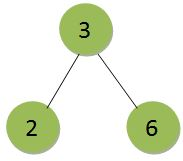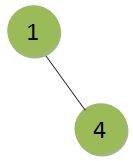Related Articles

# Generate two BSTs from the given array such that maximum height among them is minimum

• Difficulty Level : Easy
• Last Updated : 04 Oct, 2021

Given an array of n integers where n is greater than 1, the task is to make two Binary Search Tree from the given array (in any order) such that the maximum height among the two trees is minimum possible and print the maximum height.
Examples:

Input: arr[] = {1, 2, 3, 4, 6}
Output:Input: arr[] = { 74, 25, 23, 1, 65, 2, 8, 99 }
Output:

Approach: The aim is to minimize the height of the maximum height binary search tree and to do so we need to divide the array elements equally among both the trees. And since the order doesn’t matter, we can easily choose any element for the first or second binary tree. Now, to minimize the height of the two tree, the trees will have to be almost complete and as equal in heights as possible. And the maximum (minimized) height will be log(n/2) or log(n/2 + 1).
Below is the implementation of the above approach:

## C++

 `// C++ implementation of the approach``#include ``using` `namespace` `std;` `// Function to calculate the log()``int` `cal_log(``int` `n)``{``    ``int` `ans = 0;` `    ``while` `(n) {``        ``n /= 2;``        ``ans++;``    ``}` `    ``return` `ans;``}` `// Function to return the maximum``// height of the tree``int` `maximumHeight(``int` `n, ``int` `arr[])``{``    ``int` `level = cal_log(n / 2 + n % 2);``    ``return` `(level - 1);``}` `// Driven code``int` `main()``{``    ``int` `arr[] = { 1, 2, 3, 4, 6 };``    ``int` `n = ``sizeof``(arr) / ``sizeof``(arr);``    ``cout << maximumHeight(n, arr);` `    ``return` `0;``}`

## Java

 `// Java implementation of the approach``import` `java.io.*;` `class` `GFG``{` `// Function to calculate the log()``static` `int` `cal_log(``int` `n)``{``    ``int` `ans = ``0``;` `    ``while` `(n > ``0``)``    ``{``        ``n /= ``2``;``        ``ans++;``    ``}` `    ``return` `ans;``}` `// Function to return the maximum``// height of the tree``static` `int` `maximumHeight(``int` `n, ``int` `arr[])``{``    ``int` `level = cal_log(n / ``2` `+ n % ``2``);``    ``return` `(level - ``1``);``}` `// Driver code``public` `static` `void` `main (String[] args)``{``    ``int` `arr[] = { ``1``, ``2``, ``3``, ``4``, ``6` `};``    ``int` `n =arr.length;``    ``System.out.print( maximumHeight(n, arr));``}``}` `// This code is contributed by anuj_67..`

## C#

 `// C# implementation of the approach``using` `System;` `class` `GFG``{` `// Function to calculate the log()``static` `int` `cal_log(``int` `n)``{``    ``int` `ans = 0;` `    ``while` `(n > 0)``    ``{``        ``n /= 2;``        ``ans++;``    ``}` `    ``return` `ans;``}` `// Function to return the maximum``// height of the tree``static` `int` `maximumHeight(``int` `n, ``int` `[]arr)``{``    ``int` `level = cal_log(n / 2 + n % 2);``    ``return` `(level - 1);``}` `// Driver code``public` `static` `void` `Main ()``{``    ``int` `[]arr = { 1, 2, 3, 4, 6 };``    ``int` `n =arr.Length;``    ``Console.WriteLine( maximumHeight(n, arr));``}``}` `// This code is contributed by anuj_67..`

## Python3

 `# Python implementation of the approach` `# Function to calculate the log()``def` `cal_log(n):``    ``ans ``=` `0``;` `    ``while` `(n):``        ``n ``/``/``=` `2``;``        ``ans``+``=``1``;`  `    ``return` `ans;`  `# Function to return the maximum``# height of the tree``def` `maximumHeight(n, arr):``    ``level ``=` `cal_log(n ``/``/` `2` `+` `n ``%` `2``);``    ``return` `(level ``-` `1``);`  `# Driver code``arr ``=` `[ ``1``, ``2``, ``3``, ``4``, ``6` `];``n ``=` `len``(arr);``print``(maximumHeight(n, arr));` `# This code is contributed by Princi Singh`

## Javascript

 ``
Output:
`1`

Time Complexity: O(log(N))
Auxiliary Space: O(1)

Attention reader! Don’t stop learning now. Get hold of all the important DSA concepts with the DSA Self Paced Course at a student-friendly price and become industry ready.  To complete your preparation from learning a language to DS Algo and many more,  please refer Complete Interview Preparation Course.

In case you wish to attend live classes with experts, please refer DSA Live Classes for Working Professionals and Competitive Programming Live for Students.

My Personal Notes arrow_drop_up